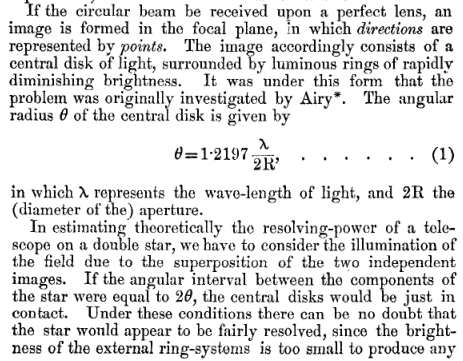# Angular Resolution Calculator

### 2021

This calculator determines the diffraction limited resolution of a camera using the Rayleigh Criterion. For a lens stopped down to a large F-number, this is a reasonable estimate, but resolution will be limited by other factors such as spherical aberation in the lens when shooting wide open. In particular, this is calculator is useful for remote imaging systems and stopped-down large format cameras.

The Rayleigh Criterion specifies the minimum angular seperation of two point sources of light such that they can be resolved as distinct objects. The equation is based on the fact that point sources of light in a diffraction limited optical system will produce Airy disks. Rayleigh's original 1879 paper describes it as:Excerpt from "Resolving, or Separating, Power of Optical Instruments"

Spatial resolution can be calculated using a small angle approximation. The default numbers below are for GeoEye-1 with 550nm green light, and the equation predicts exactly the advertised ground resolution: 0.41m. (sensor size is assumed based on 15x15km single point scenes )Wavelength to Color Chart